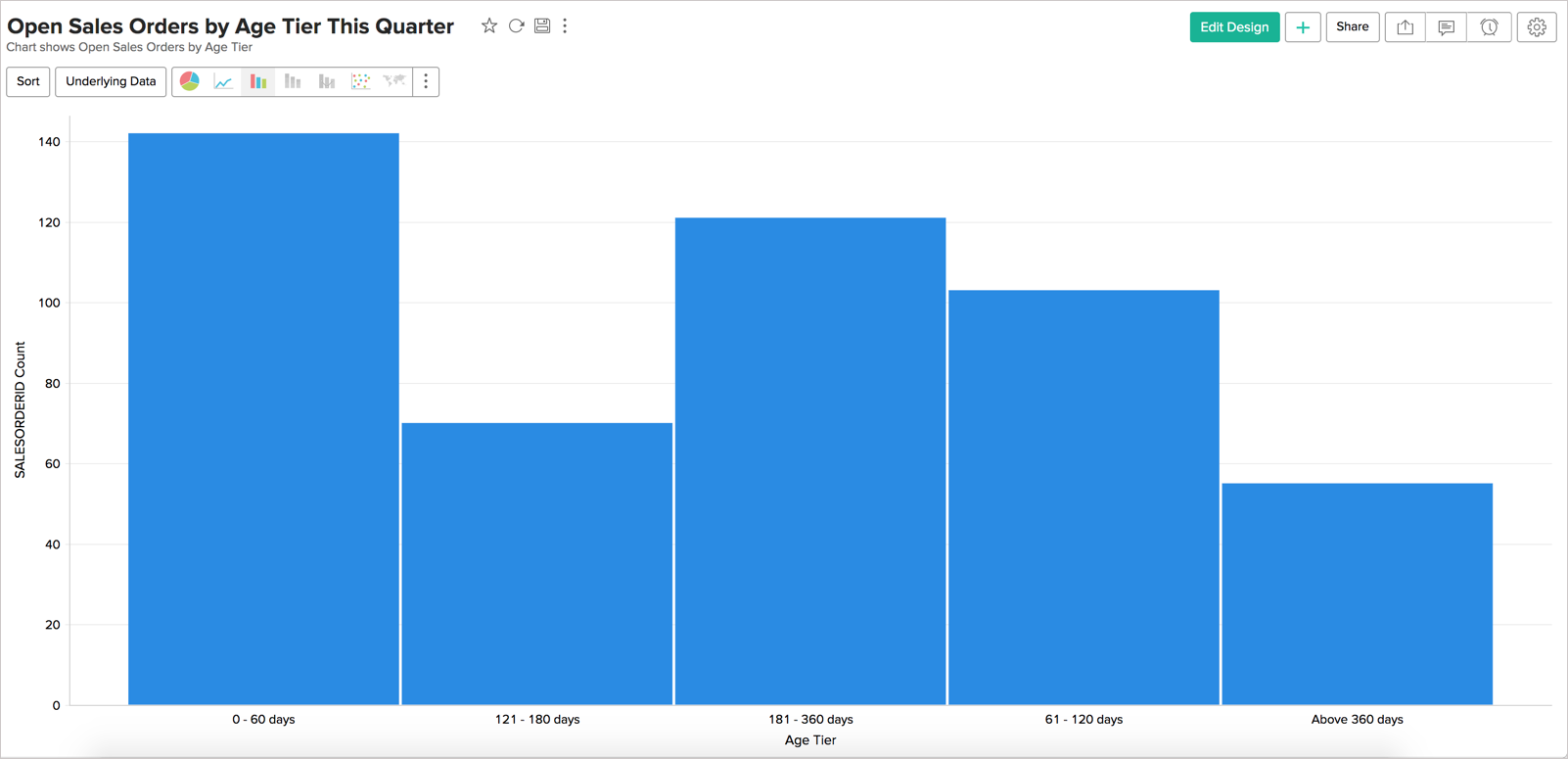# Histogram Chart

Histogram Chart is a special form of bar chart where the values are plotted over continuous rather than discrete categories and useful to plot frequency distributions.

## Creating a Histogram chart

Follow the below steps to create a Histogram chart.

1. Open the table over which you want to create the chart.
2. Click New icon and then click New Chart View.
3. In the chart editor that opens, drop columns as given below.
• X-Axis - Drop the column to categorize the data.
• Y-Axis - Drop the metric column.
4. Click the Click here to Generate Graph button. A line bar chart will be created.
5. Change Chart Type to Bar > Histogram.## Possible combinations for creating Histogram Charts

The following combinations of column types are possible when creating Histogram charts.

 X axis Y axis Color Text Tooltip Case 1 Dimension Aggregate/Optional - - - Case 2 Optional Aggregate - - - Case 3 Aggregate/Optional Dimension - - - Case 4 Aggregate Optional - - -
• Dimension: A column with Actual Value function applied over it. For numeric column, Actual value will be divided into Actual Measure, Actual Dimension, and Actual Range.
• Aggregate: A column with an aggregate function applied (sum, average, count, etc) applied over it. This also includes aggregate formula columns.